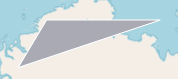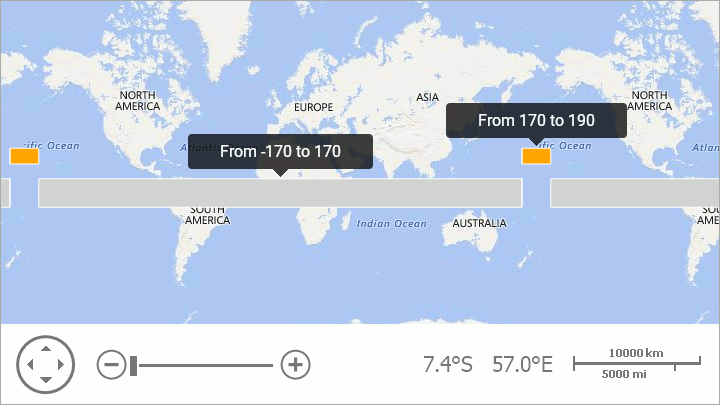21.1
20.2
20.1
19.2
19.1
18.2
18.1
17.2

# MapPolygon Class

The class used to draw a polygon on a map.

Namespace: DevExpress.XtraMap

Assembly: DevExpress.XtraMap.v21.1.dll

## Declaration

``````public class MapPolygon :
MapShape,
ISupportCoordPoints,
IPolygonCore,
IPointContainerCore,
IEditableItem,
ISimplifiableItem``````

The following members accept/return MapPolygon objects:

## Remarks

The following image shows an example of a map polygon object.Note that, a map shape should fulfill the following condition to cross the 180th meridian: one or several points’ longitudes should exceed the 180 (-180) limit:

``````MapPolygon polygon = new MapPolygon();
polygon.Points.Add(new GeoPoint(10, -170));
polygon.Points.Add(new GeoPoint(10, 170));
polygon.Points.Add(new GeoPoint(-10, 170));
polygon.Points.Add(new GeoPoint(-10, -170));
vectorItemStorage.Items.Add(polygon);

// Point longitudes enclose the 180 meridian.
MapPolygon polygonCross180 = New MapPolygon();
polygonCross180.Fill = Color.Orange;
polygonCross180.Points.Add(new GeoPoint(30, 190));
polygonCross180.Points.Add(new GeoPoint(30, 170));
polygonCross180.Points.Add(new GeoPoint(20, 170));
polygonCross180.Points.Add(new GeoPoint(20, 190));
vectorItemStorage.Items.Add(polygon180);
``````

The code above produces the following image:## Example

``````var polygon = new MapPolygon();
polygon.Points.AddRange(new GeoPoint[] {
new GeoPoint(70,80),
new GeoPoint(75,90),
new GeoPoint(75,130)
});
ItemStorage.Items.Add(polygon);
``````

See Also